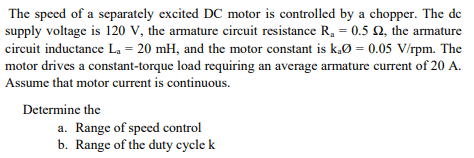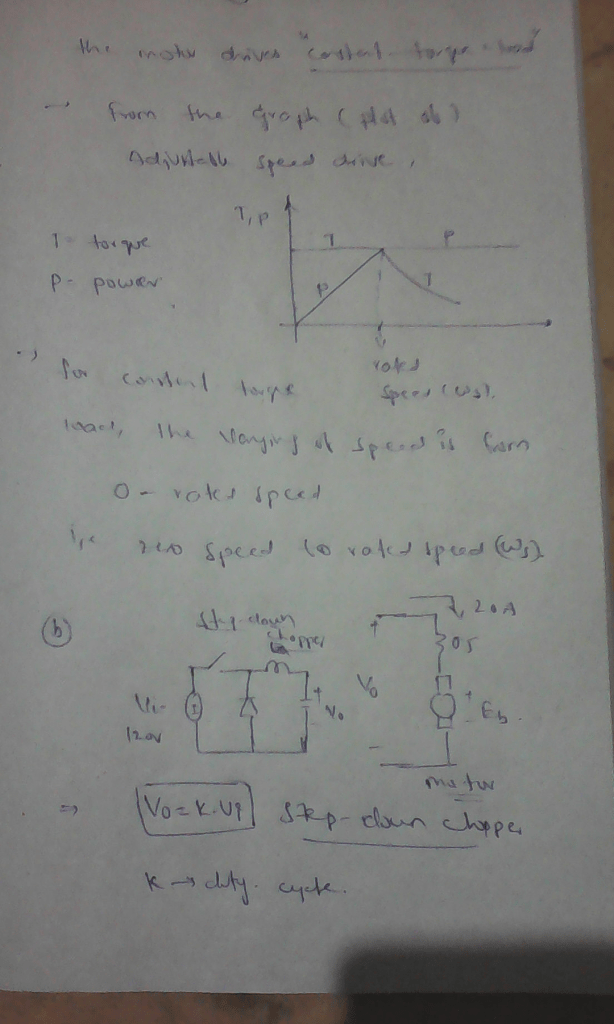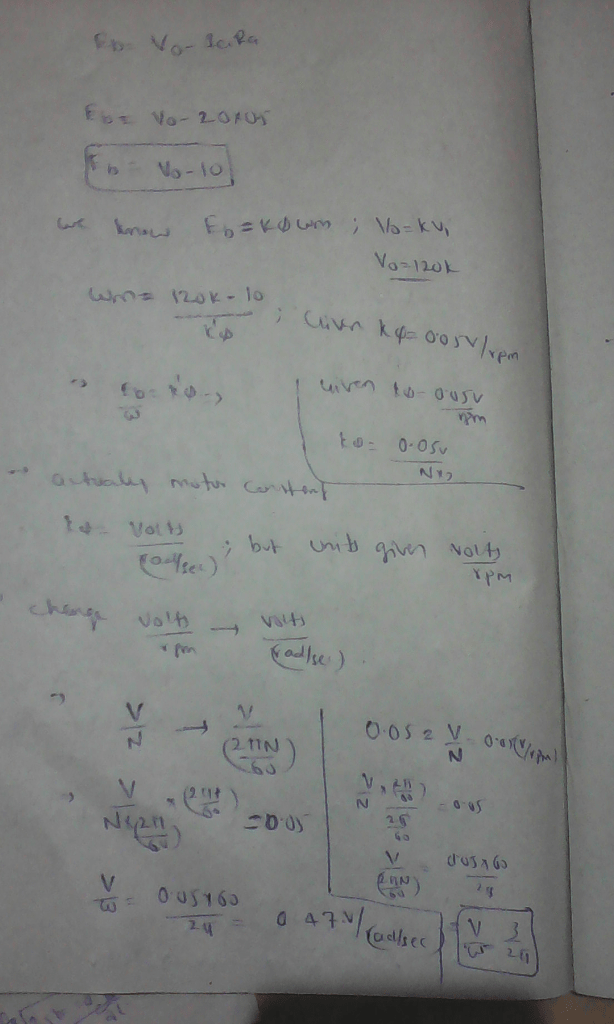# The speed of a separately excited DC motor is controlled by a chopper. The dc supply voltage is 120 V, the armature circuit resistance R,-0.5 Ω, the armature circuit inductance La 20 mH, and the motor constant is ka0 0.05 V/rpm. The Assume that motor current is continuous. Determine the a. Range of speed control b. Range of the duty cycle k

Question-AnswerCategory: Electrical EngineeringThe speed of a separately excited DC motor is controlled by a chopper. The dc supply voltage is 120 V, the armature circuit resistance R,-0.5 Ω, the armature circuit inductance La 20 mH, and the motor constant is ka0 0.05 V/rpm. The Assume that motor current is continuous. Determine the a. Range of speed control b. Range of the duty cycle k

The speed of a separately excited DC motor is controlled by a chopper. The dc supply voltage is 120 V, the armature circuit resistance R,-0.5 Ω, the armature circuit inductance La 20 mH, and the motor constant is ka0 0.05 V/rpm. The Assume that motor current is continuous. Determine the a. Range of speed control b. Range of the duty cycle k